# [Maya C++] add control curve/spline attributeAllow user to customize a 2D curve (spline etc.) to control some parameters such as color, grading and more.

# Distributing Maya pluginsI will discuss various ways you can ship a Maya plugin to users.

# Diffusing / smoothing weight map over a triangular mesh.Showcasing simple procedures with C++ code to smooth / diffuse per vertex weights over a triangle mesh.

# Implicit surface a.k.a (signed) distance field: definitionIf I say "implicit surface" you may think of metaballs and marching cube, I'll show you there is more to it!

# Dual Quaternion Skinning with scaleDQS with scale applied on the second to last joint. Left, globally propagates until the last bone, right, scale localized to each joint.

# Transforming implicit surface (distance field) and their gradient# Transform normals given a deformation map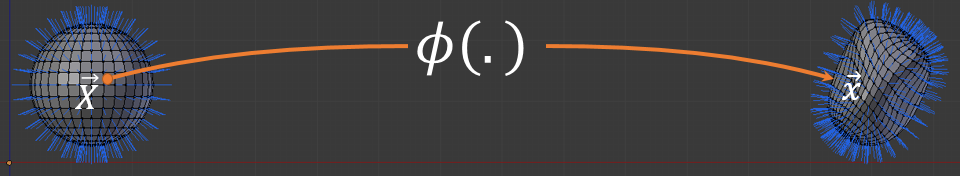# Blender 2.5 - 2.7 tutorial to animate a cylinder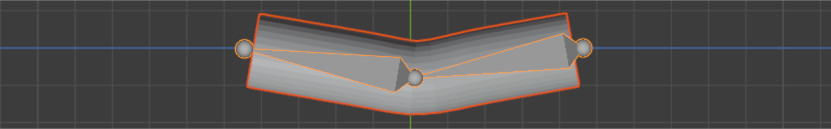# Design of a simple sphere deformer to displace a mesh model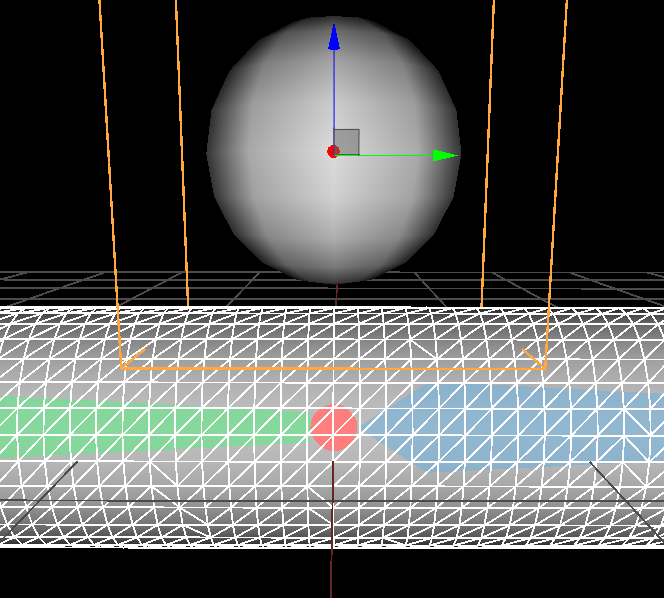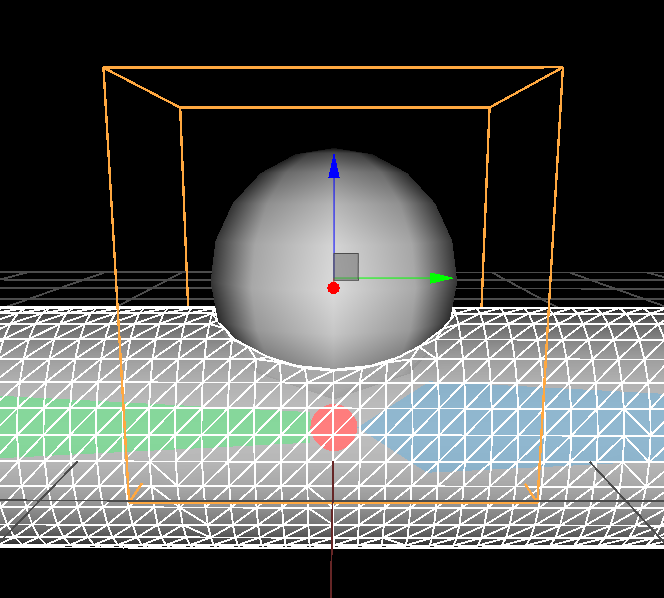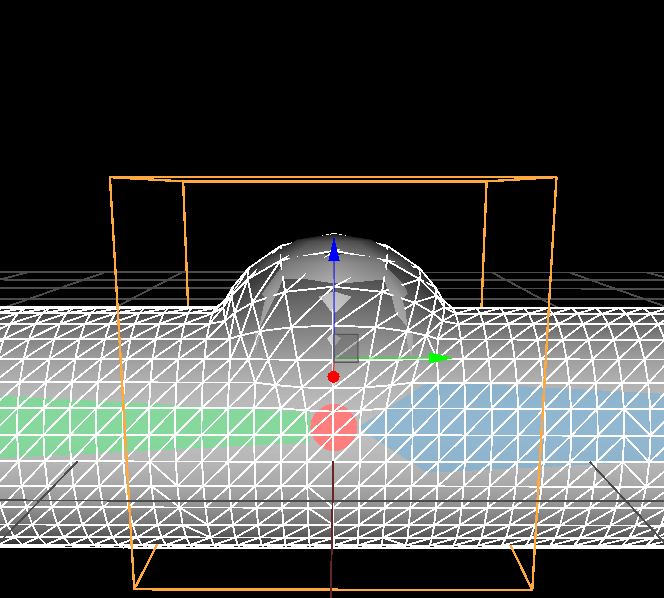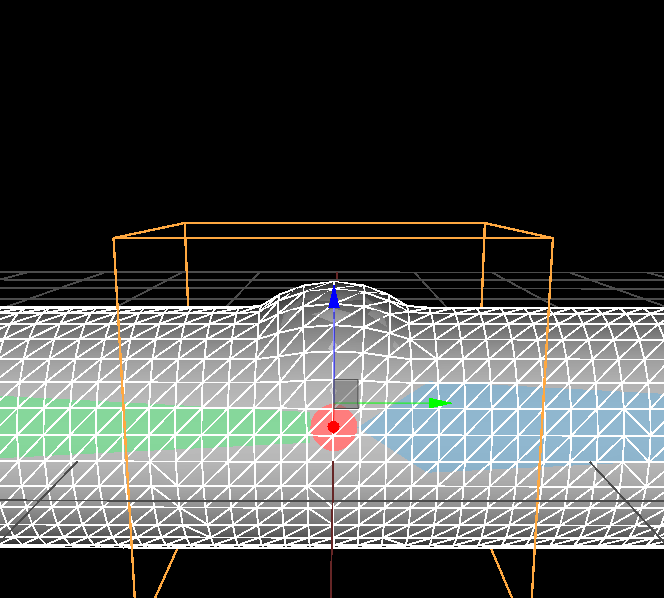# 2D biharmonic stencil a.k.a bilaplacian operatorDraft / work in progress

# Harmonic function: definitions and propertiesThis is the second part of my tutorial series on bounded harmonic functions. For a quick introduction and examples of use of harmonic functions read the first part. In this part I define harmonic functions and their properties. This is the hard part with a lot of mathematics. But it's a mandatory step to understand how harmonic functions work. This will allow you to apply them in a broader context and understand many scientific papers relying on these. In addition this will be my entry point to introduce Finite Element Method in future posts. So hang on it's worth it!

# How to generate bounded harmonic weights on a regular grid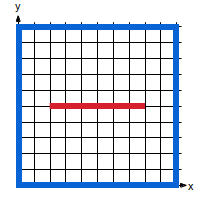(a)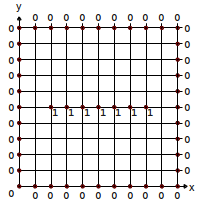(b)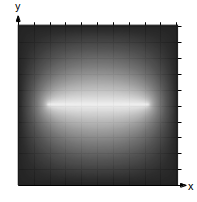(c)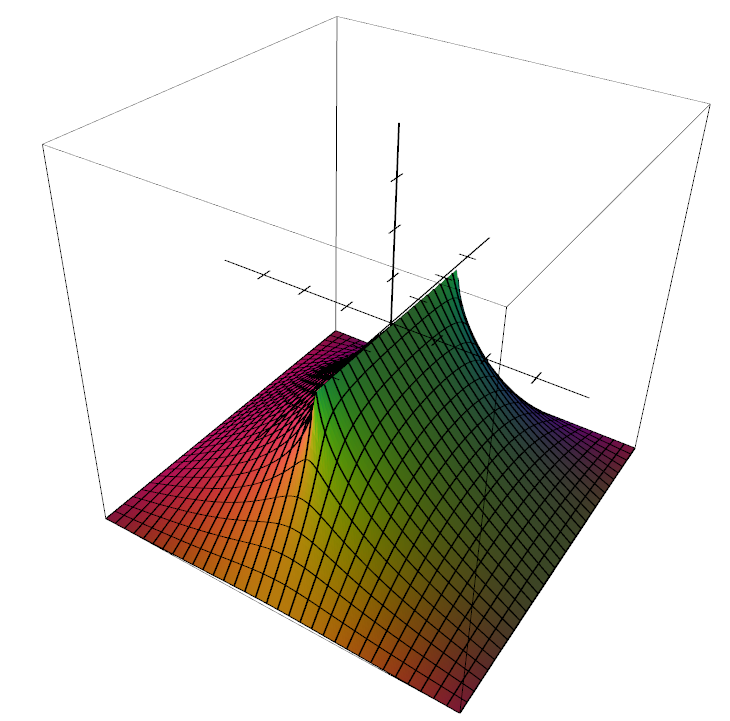(d)
 Generating an height-map  is a good example to demonstrate how useful harmonic functions can be. In (a) user can stroke lines corresponding to different heights. In (b) we associate a height where the strokes lies. In (c) we compute the rest of the values automatically (black is zero, white is one). (d) is a 3D view of the heightmap in (c). It is a 2D function $$f: \mathbb R^2 \rightarrow \mathbb R$$ which returns the height. The tutorial explains how to compute the unknown heights and ensure $$f$$ is an harmonic function.

## Summary

In this tutorial I give the basics to be able to compute harmonic functions $$f: \mathbb R^n \rightarrow \mathbb R$$ over a regular grid/texture (in 1D, 2D or 3D). All you need is to set a few values on the grid that define a closed region (see figure above), then the remaining values are automatically computed using the so-called Laplace operator and solving a linear system of equations. After a quick and "mathless" presentation of an easy way to implement this in C++, I will explain the mathematical jargon. This tutorial is the first of series which will lead to more complicated techniques like Finite Element Methods (FEM).

# Dual Quaternions skinning tutorial and C++ codes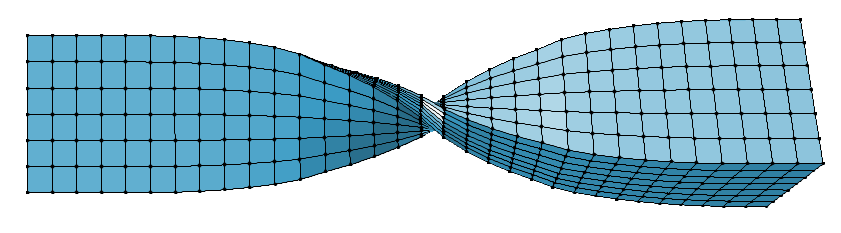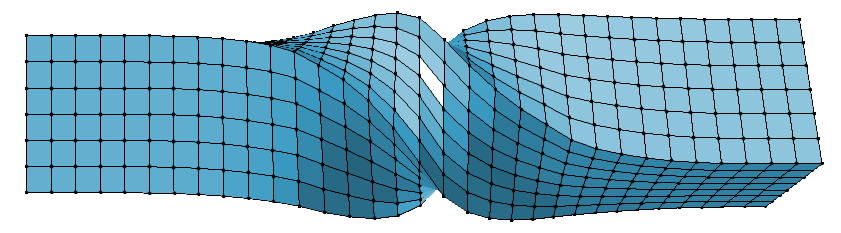In this entry I provide [C++ codes ] to deform a mesh with the famous Dual Quaternion Skinning (DQS) deformer.

# Convert implicit surface defined with global support to compact supportIn mathematics functions are sometime characterized as compactly or globally supported. I will explain what it means and how to convert a function from global to compact support in the context of implicit surface modeling (i.e. boolean modeling with implicit surfaces as objects).

# Compute Harmonic weights on a triangular meshHere I describe the discreet Laplace-Beltrami operator for a triangle mesh and how to derive the Laplacian matrix for that mesh. Then I provide [C++ code ] to compute harmonic weights over a triangular mesh by solving the Laplace equation.

# Recipe for implicit surface reconstruction with HRBF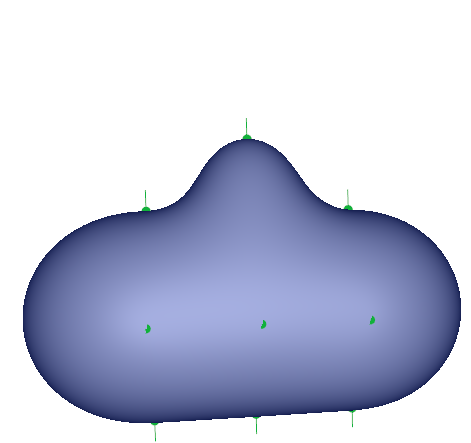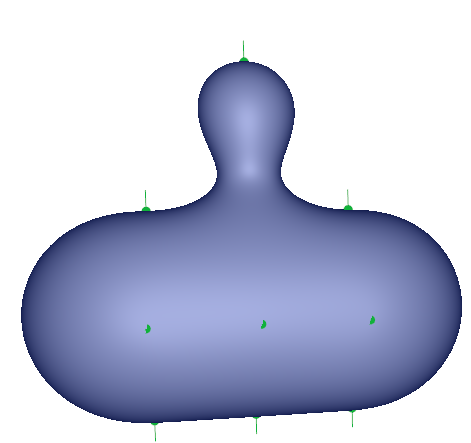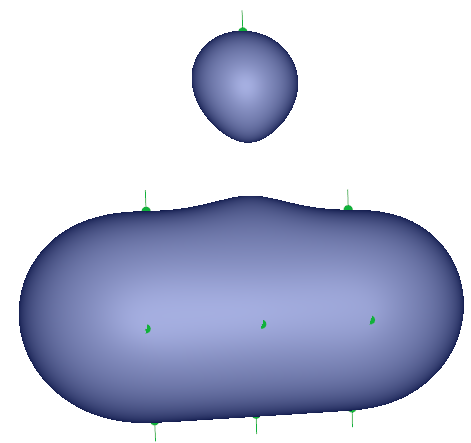In this entry I'll explain and give code for the easiest method I know to reconstruct a surface represented by a scalar field $$f: \mathbb R^3 \rightarrow \mathbb R$$ by interpolating a set of point with their normals. The method is called Hermite Radial Basis Function (HRBF) interpolation. The audience aimed is anyone with some basic knowledge about implicit surfaces (which people often know as metaballs and render with the marching cube algorithm)

•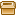HRBF core sources ] [HRBF toy app sources ] [HRBF toy app binaries
•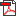maths details ]  [maths summary ] [tex sources (FR) (EN) ]